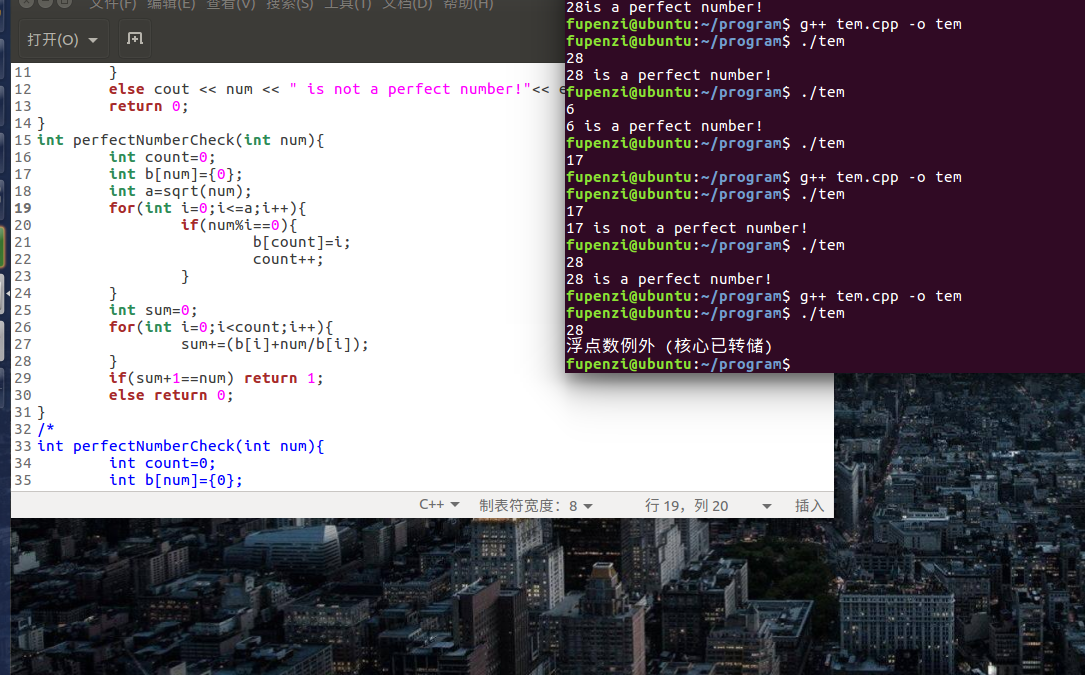# 整数求因数算法

#include<iostream>
#include<cmath>
using namespace std;

int perfectNumberCheck(int);
int main(){
int num;
cin >> num;
if(perfectNumberCheck(num)){
cout << num << " is a perfect number!"<< endl;
}
else cout << num << " is not a perfect number!"<< endl;
return 0;
}
int perfectNumberCheck(int num){
int count=0;
int b[num]={0};
int a=sqrt(num);
for(int i=2;i<=a;i++){
if(num%i==0){
b[count]=i;
count++;
}
}
int sum=0;
for(int i=0;i<count;i++){
sum+=(b[i]+num/b[i]);
}
if(sum+1==num) return 1;
else return 0;
}
/*
int perfectNumberCheck(int num){
int count=0;
int b[num]={0};
for(int i=2;i*i<=num;i++){ //直接使用i*i，可以避免使用开平方运算
if(num%i==0){
b[count]=i;
count++;
}
}
int sum=0;
for(int i=0;i<count;i++){
sum+=(b[i]+num/b[i]);
}
if(sum+1==num) return 1;
else return 0;
}
*/09-18115104-051万+
09-19
02-238658
08-29365
02-241703
04-252626
06-03771
07-223440
01-045919
09-041922
07-291万+
08-093082
06-15
06-059662
06-012775
12-054276
09-151万+